# Dragging problem

This article discusses a scenario/arrangement whose statics/dynamics/kinematics can be understood using the ideas of classical mechanics.
View other mechanics scenarios

## The scenario

Suppose a block$m$ rests on a fixed horizontal floor. The limiting coefficient of static friction between$m$ and the floor is$\mu_s$ and the coefficient of kinetic friction is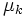$\mu_k$. A force$F$ is applied on the block in a diagonal direction at an angle$\theta$ with the horizontal. The questions are as follows:

• For a given angle$\theta$, what is the minimum magnitude of force needed to get the block to start sliding, and how is the acceleration of the block given as a function of$F$?
• For what value of$\theta$ is the minimum magnitude of force needed to get the block to start sliding as low as possible, and what is this minimum magnitude of force?
• For what value of$\theta$ is the minimum magnitude of force needed to get the block to keep sliding as low as possible, and what is this minimum magnitude of force?

## Basic components of force diagram

Force (letter) Nature of force Condition for existence Magnitude Direction$F$ external force being applied given to us that it's being applied$F$ angle$\theta$ with horizontal. The horizontal component is$F \cos \theta$ and the vertical component is$F \sin \theta$.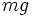$mg$ gravitational force unconditional$mg$ vertical, downward$N$ normal force assuming that$F \sin \theta \le mg$, otherwise the block will lift off the floor. adjusts so that there is no net force in the vertical direction vertical, upward$f_s$ static friction no sliding adjusts so that there is no net force in the horizontal direction horizontal, opposite to horizontal component of$F$$f_k$ kinetic friction sliding$\mu_kN$ horizontal, opposite to direction of slipping. Assuming initially at rest, direction of slipping = horizontal component of$F$, so this is opposite to horizontal component of$F$.

### Vertical components

Taking components in the vertical direction, and assuming no acceleration in the vertical direction (because the block does not lift off), we get: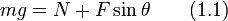$mg = N + F \sin \theta \qquad (1.1)$

### Horizontal components and solution assuming no sliding

If there is no sliding, we get: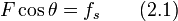$F \cos \theta = f_s \qquad (2.1)$

We also have:$f_s \le \mu_sN \qquad (2.2)$

Plugging in$N$ from (1.1) into (2.2) and plugging$f_s$ from this into (2.1) gives: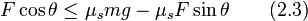$F \cos \theta \le \mu_smg - \mu_sF\sin \theta \qquad (2.3)$

This simplifies to: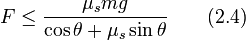$F \le \frac{\mu_smg}{\cos \theta + \mu_s \sin \theta} \qquad (2.4)$

This is a necessary and sufficient condition for there to be no sliding assuming the block was initially at rest.

### Horizontal components and solution assuming sliding

If there is sliding along the horizontal component of the direction of$F$, denote by$a$ the acceleration along the horizontal component of the direction of$F$. We get: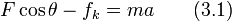$F \cos \theta - f_k = ma \qquad (3.1)$

We also have:$f_k = \mu_kN \qquad (3.2)$

Plugging in$N$ from (1.1) into (3.2) and plugging$f_k$ from this into (3.1) gives: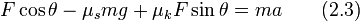$F \cos \theta - \mu_smg + \mu_kF\sin \theta = ma\qquad (2.3)$

This simplifies to:$a = \frac{F(\cos \theta + \mu_k \sin \theta)}{m} - \mu_sg \qquad (2.4)$

## Angle needed to minimize the minimum force needed to start sliding

As noted in equation (2.4), the minimum force needed to start sliding is: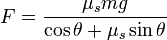$F = \frac{\mu_smg}{\cos \theta + \mu_s \sin \theta} \qquad$

The numerator is constant, so to minimize this is equvalent to maximizing the denominator, for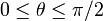$0 \le \theta \le \pi/2$. In other words, we need to maximize: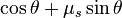$\cos \theta + \mu_s \sin \theta$

Using either differential calculus or basic trigonometry or the Cauchy-Schwarz inequality, we get that the maximum occurs when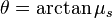$\theta = \arctan \mu_s$, and the value of the maximum is$\sqrt{1 + \mu_s^2}$, so that the minimum possible value of the minimum force needed is:$F = \frac{\mu_smg}{\sqrt{1 + \mu_s^2}}$